JEE  >  NCERT Solution - Relations and Functions (Ex - 2.1)

# NCERT Solution - Relations and Functions (Ex - 2.1) Notes | Study Additional Documents and Tests for JEE - JEE

## Document Description: NCERT Solution - Relations and Functions (Ex - 2.1) for JEE 2022 is part of Documents for Additional Documents and Tests for JEE preparation. The notes and questions for NCERT Solution - Relations and Functions (Ex - 2.1) have been prepared according to the JEE exam syllabus. Information about NCERT Solution - Relations and Functions (Ex - 2.1) covers topics like and NCERT Solution - Relations and Functions (Ex - 2.1) Example, for JEE 2022 Exam. Find important definitions, questions, notes, meanings, examples, exercises and tests below for NCERT Solution - Relations and Functions (Ex - 2.1).

Introduction of NCERT Solution - Relations and Functions (Ex - 2.1) in English is available as part of our Additional Documents and Tests for JEE for JEE & NCERT Solution - Relations and Functions (Ex - 2.1) in Hindi for Additional Documents and Tests for JEE course. Download more important topics related with Documents, notes, lectures and mock test series for JEE Exam by signing up for free. JEE: NCERT Solution - Relations and Functions (Ex - 2.1) Notes | Study Additional Documents and Tests for JEE - JEE
 1 Crore+ students have signed up on EduRev. Have you?

NCERT QUESTION

(Ex - 2.1)

Ques 1: If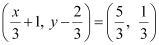, find the values of x and y

Ans: It is given that.

Since the ordered pairs are equal, the corresponding elements will also be equal.

Therefore,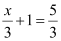and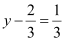.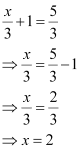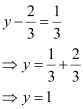x = 2 and y = 1

Ques 2: If the set A has 3 elements and the set B = {3, 4, 5}, then find the number of elements in (A × B)?

Ans: It is given that set A has 3 elements and the elements of set B are 3, 4, and 5.

⇒ Number of elements in set B = 3

Number of elements in (A × B)

= (Number of elements in A) × (Number of elements in B)

= 3 × 3 = 9

Thus, the number of elements in (A × B) is 9.

Ques 3: If G = {7, 8} and H = {5, 4, 2}, find G × H and H × G.

Ans: G = {7, 8} and H = {5, 4, 2}

We know that the Cartesian product P × Q of two non-empty sets P and Q is defined as

P × Q = {(p, q): p∈ P, q ∈ Q}

∴G × H = {(7, 5), (7, 4), (7, 2), (8, 5), (8, 4), (8, 2)}

H × G = {(5, 7), (5, 8), (4, 7), (4, 8), (2, 7), (2, 8)}

Ques 4: State whether each of the following statement are true or false. If the statement is false, rewrite the given statement correctly.

(i) If P = {m, n} and Q = {n, m}, then P × Q = {(m, n), (n, m)}.

(ii) If A and B are non-empty sets, then A × B is a non-empty set of ordered pairs (x, y) such that x ∈ A and y ∈ B.

(iii) If A = {1, 2}, B = {3, 4}, then A × (B ∩ Φ) = Φ.

Ans: (i) False

If P = {m, n} and Q = {n, m}, then

P × Q = {(m, m), (m, n), (n, m), (n, n)}

(ii) True

(iii) True

Ques 5: If A = {–1, 1}, find A × A × A.

Ans: It is known that for any non-empty set A, A × A × A is defined as

A × A × A = {(a, b, c): a, b, c ∈ A}

It is given that A = {–1, 1}

∴ A × A × A = {(–1, –1, –1), (–1, –1, 1), (–1, 1, –1), (–1, 1, 1),

(1, –1, –1), (1, –1, 1), (1, 1, –1), (1, 1, 1)}

Ques 6: If A × B = {(a, x), (a, y), (b, x), (b, y)}. Find A and B.

Ans: It is given that A × B = {(a, x), (a, y), (b, x), (b, y)}

We know that the Cartesian product of two non-empty sets P and Q is defined as P × Q = {(p, q): p ∈ P, q ∈ Q}

∴ A is the set of all first elements and B is the set of all second elements.

Thus, A = {a, b} and B = {x, y}

Ques 7: Let A = {1, 2}, B = {1, 2, 3, 4}, C = {5, 6} and D = {5, 6, 7, 8}. Verify that

(i) A × (B ∩ C) = (A × B) ∩ (A × C)

(ii) A × C is a subset of B × D

Ans: (i) To verify: A × (B ∩ C) = (A × B) ∩ (A × C)

We have B ∩ C = {1, 2, 3, 4} ∩ {5, 6} = Φ

∴L.H.S. = A × (B ∩ C) = A × Φ = Φ

A × B = {(1, 1), (1, 2), (1, 3), (1, 4), (2, 1), (2, 2), (2, 3), (2, 4)}

A × C = {(1, 5), (1, 6), (2, 5), (2, 6)}

∴ R.H.S. = (A × B) ∩ (A × C) = Φ

∴L.H.S. = R.H.S

Hence, A × (B ∩ C) = (A × B) ∩ (A × C)

(ii) To verify: A × C is a subset of B × D

A × C = {(1, 5), (1, 6), (2, 5), (2, 6)}

B × D = {(1, 5), (1, 6), (1, 7), (1, 8), (2, 5), (2, 6), (2, 7), (2, 8), (3, 5), (3, 6), (3, 7), (3, 8), (4, 5), (4, 6), (4, 7), (4, 8)}

We can observe that all the elements of set A × C are the elements of set B × D.

Therefore, A × C is a subset of B × D.

Ques 8: Let A = {1, 2} and B = {3, 4}. Write A × B. How many subsets will A × B have? List them.

Ans: A = {1, 2} and B = {3, 4}

∴A × B = {(1, 3), (1, 4), (2, 3), (2, 4)}

n(A × B) = 4

We know that if C is a set with n(C) = m, then n[P(C)] = 2m.

Therefore, the set A × B has 24 = 16 subsets. These are

Φ, {(1, 3)}, {(1, 4)}, {(2, 3)}, {(2, 4)}, {(1, 3), (1, 4)}, {(1, 3), (2, 3)},

{(1, 3), (2, 4)}, {(1, 4), (2, 3)}, {(1, 4), (2, 4)}, {(2, 3), (2, 4)},

{(1, 3), (1, 4), (2, 3)}, {(1, 3), (1, 4), (2, 4)}, {(1, 3), (2, 3), (2, 4)},

{(1, 4), (2, 3), (2, 4)}, {(1, 3), (1, 4), (2, 3), (2, 4)}

Ques 9: Let A and B be two sets such that n(A) = 3 and n (B) = 2. If (x, 1), (y, 2), (z, 1) are in A × B, find A and B, where x, y and z are distinct elements.

Ans: It is given that n(A) = 3 and n(B) = 2; and (x, 1), (y, 2), (z, 1) are in A × B.

We know that A = Set of first elements of the ordered pair elements of A × B

B = Set of second elements of the ordered pair elements of A × B.

x, y, and z are the elements of A; and 1 and 2 are the elements of B.

Since n(A) = 3 and n(B) = 2, it is clear that A = {x, y, z} and B = {1, 2}.

Ques 10: The Cartesian product A × A has 9 elements among which are found (–1, 0) and (0, 1). Find the set A and the remaining elements of A × A.

Ans: We know that if n(A) = p and n(B) = q, then n(A × B) = pq.

n(A × A) = n(A) × n(A)

It is given that n(A × A) = 9

n(A) × n(A) = 9

n(A) = 3

The ordered pairs (–1, 0) and (0, 1) are two of the nine elements of A × A.

We know that A × A = {(a, a): a ∈ A}. Therefore, –1, 0, and 1 are elements of A.

Since n(A) = 3, it is clear that A = {–1, 0, 1}.

The remaining elements of set A × A are (–1, –1), (–1, 1), (0, –1), (0, 0),

(1, –1), (1, 0), and (1, 1)

The document NCERT Solution - Relations and Functions (Ex - 2.1) Notes | Study Additional Documents and Tests for JEE - JEE is a part of the JEE Course Additional Documents and Tests for JEE.
All you need of JEE at this link: JEE

## Additional Documents and Tests for JEE

11 videos|159 docs|14 tests
 Use Code STAYHOME200 and get INR 200 additional OFF

## Additional Documents and Tests for JEE

11 videos|159 docs|14 tests

Track your progress, build streaks, highlight & save important lessons and more!

,

,

,

,

,

,

,

,

,

,

,

,

,

,

,

,

,

,

,

,

,

;